# Valence Electrons Day 1 Review Electrons are the

• Slides: 23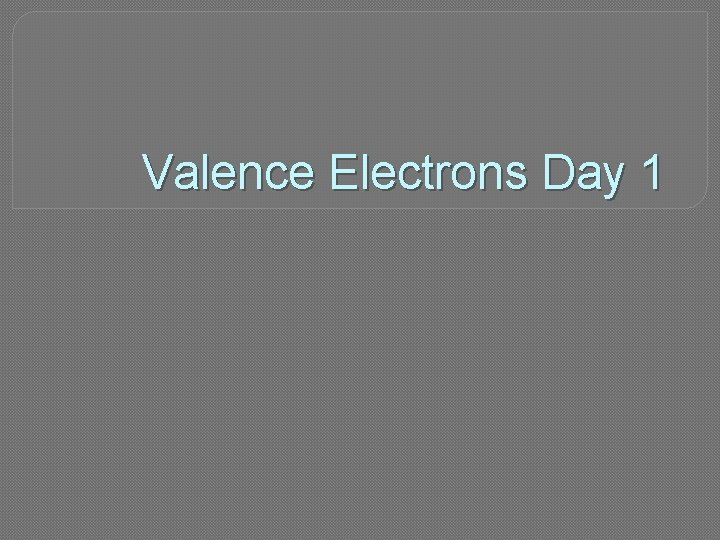Valence Electrons Day 1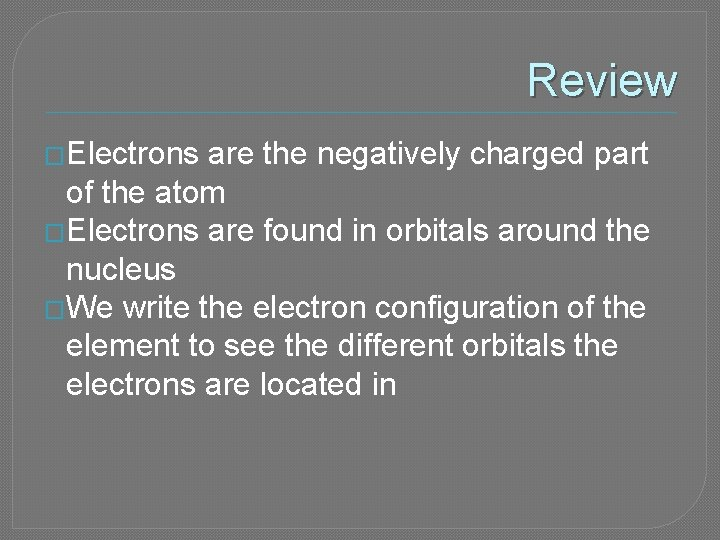Review �Electrons are the negatively charged part of the atom �Electrons are found in orbitals around the nucleus �We write the electron configuration of the element to see the different orbitals the electrons are located in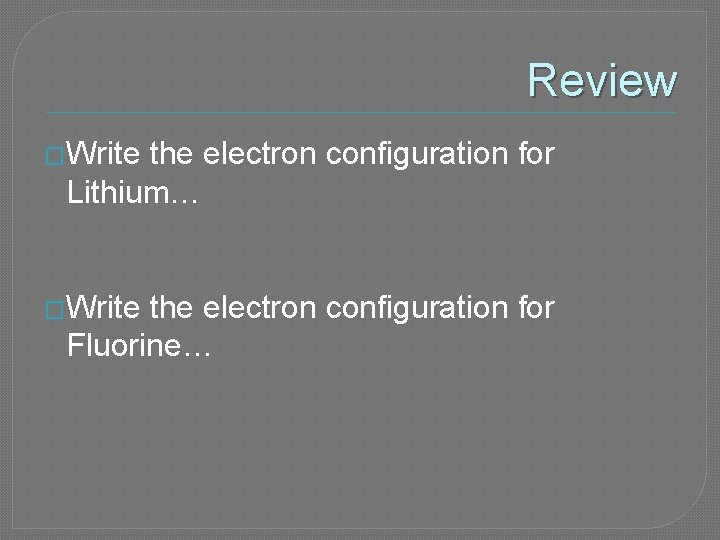Review �Write the electron configuration for Lithium… �Write the electron configuration for Fluorine…Valence electrons �The electrons in the last energy level of the element are the VALENCE electrons �The last energy level is the part of the configuration that has the largest number in front of it Lithium = 1 s 2 2 s 1 Fluorine = 1 s 2 2 s 22 p 5Valence electrons �An element can have anywhere from 1 -8 valence electrons �The number of valence electrons determines how REACTIVE the element is � 8 = totally unreactive � 1 and 7 = extremely reactive � 2 and 6 = pretty reactive � 3 -5 = only kinda reactiveHow to find valence electrons? Pull out your periodic table! You can find the valence electron of the element by seeing what group it is in…. Where are the groups on the periodic table?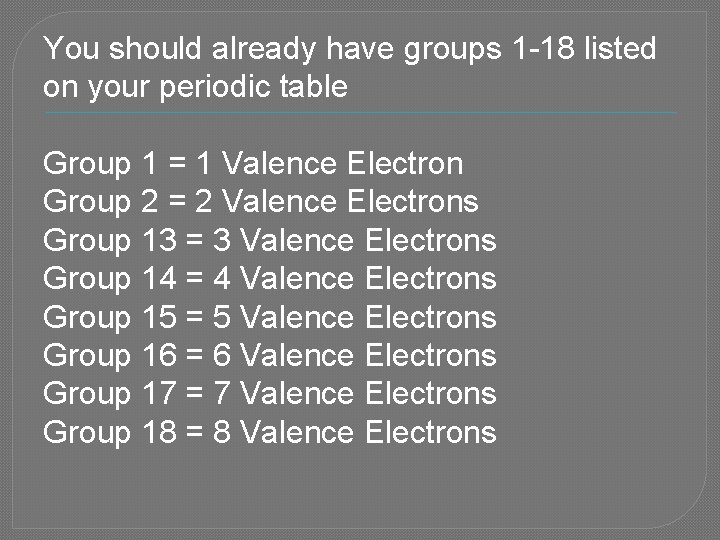You should already have groups 1 -18 listed on your periodic table Group 1 = 1 Valence Electron Group 2 = 2 Valence Electrons Group 13 = 3 Valence Electrons Group 14 = 4 Valence Electrons Group 15 = 5 Valence Electrons Group 16 = 6 Valence Electrons Group 17 = 7 Valence Electrons Group 18 = 8 Valence ElectronsWhat about groups 3 -12? �The transition metals in groups 3 -12 have their own set of rules �Don’t worry about them for nowIn - Class Practice: Due at end of hour! �Write down the following elements on a sheet of paper… next to them find out how many valence electrons they have? 1. Neon 2. Sodium 3. Aluminum 4. Calcium 5. Sulfur 6. Iodine 7. Carbon 8. Lead 9. Phosphorus 10. Krypton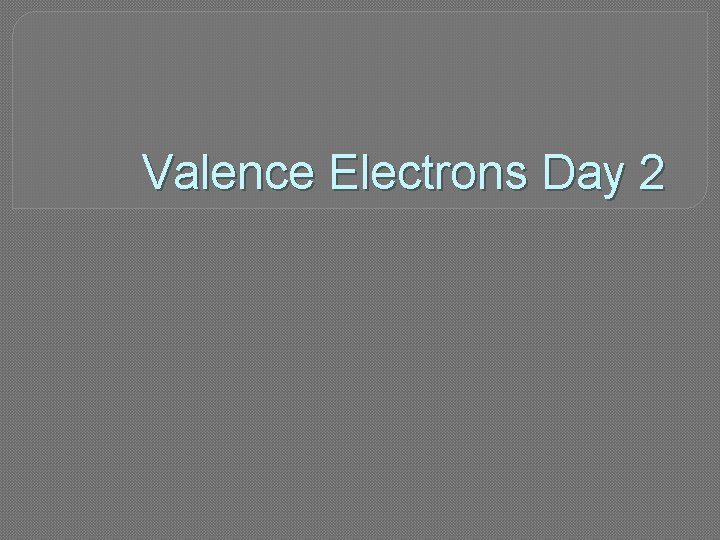Valence Electrons Day 2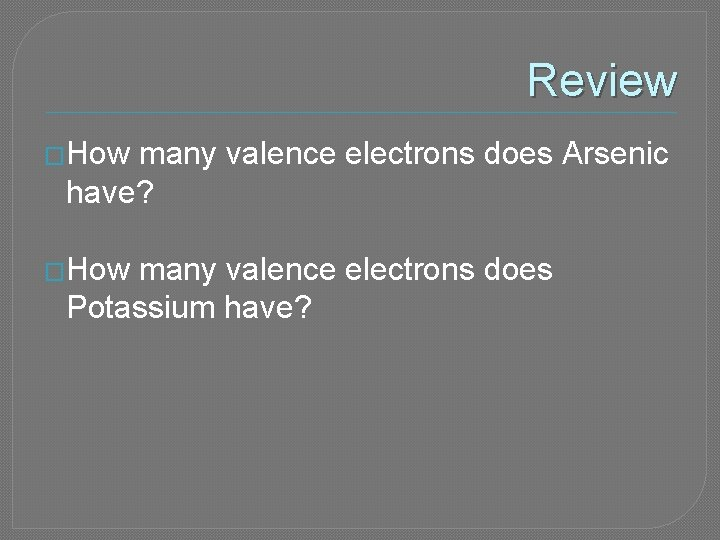Review �How many valence electrons does Arsenic have? �How many valence electrons does Potassium have?Valence Electrons �To find the number of valence electrons for the elements in groups 1, 2 and 13 -18 you look at your periodic table �Transition own rules metals (groups 3 -12) have their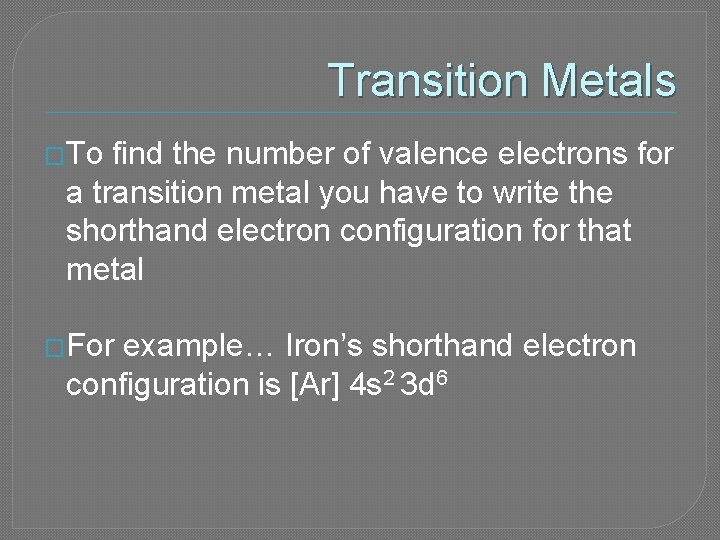Transition Metals �To find the number of valence electrons for a transition metal you have to write the shorthand electron configuration for that metal �For example… Iron’s shorthand electron configuration is [Ar] 4 s 2 3 d 6Transition Metals �Just like the regular valence electrons, we look at the highest energy level �Iron’s shorthand electron configuration is [Ar] 4 s 2 3 d 6 4 s 2 = highest energy level �Iron has 2 valence electronsTransition Metals � All transition metals will have a shorthand configuration that gives you 2 valence electrons �However, Transition Metals can act like they have anywhere from 1 -4 electronsHomework “Valence Electrons” side of worksheet For each question you should 1. Write the shorthand electron configuration 2. Write how many valence electrons each element hasValence Electrons Day 3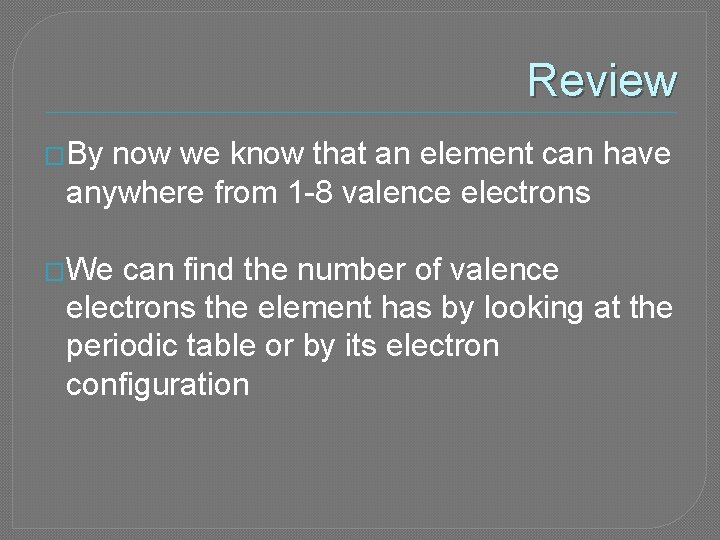Review �By now we know that an element can have anywhere from 1 -8 valence electrons �We can find the number of valence electrons the element has by looking at the periodic table or by its electron configuration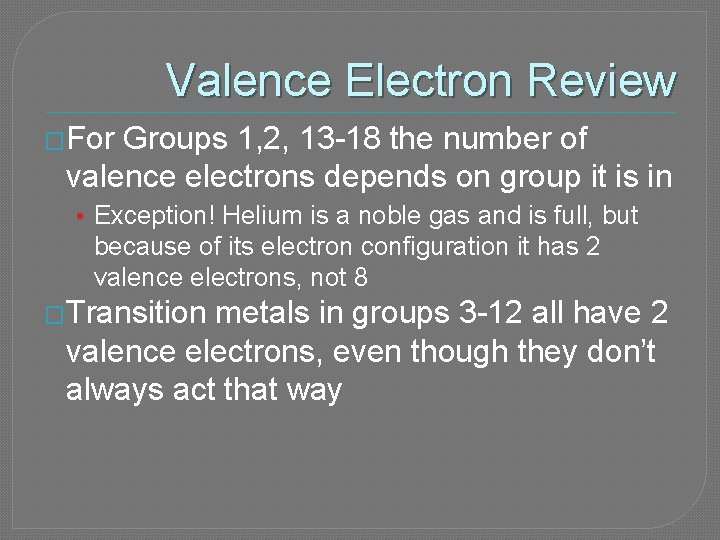Valence Electron Review �For Groups 1, 2, 13 -18 the number of valence electrons depends on group it is in • Exception! Helium is a noble gas and is full, but because of its electron configuration it has 2 valence electrons, not 8 �Transition metals in groups 3 -12 all have 2 valence electrons, even though they don’t always act that wayLewis Structures �We can draw Lewis Structures to represent the valence electrons of an element To draw a Lewis Structure 1. Put the element symbol in the middle 2. Determine how many valence electrons it has 3. Draw a dot for each electron around the symbolImportant Rules �As you are putting the dots around the symbol you start by putting one dot on each side of the symbol �No side should ever have more than 2 dots Example: Electron dot diagram for Carbon : C: C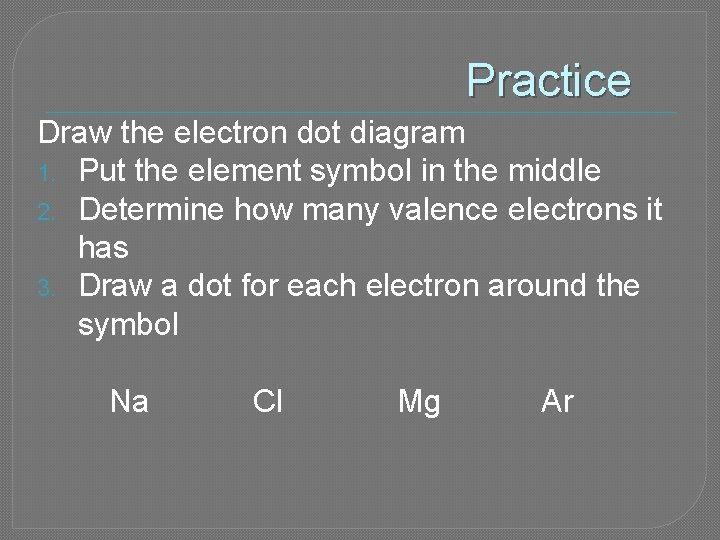Practice Draw the electron dot diagram 1. Put the element symbol in the middle 2. Determine how many valence electrons it has 3. Draw a dot for each electron around the symbol Na Cl Mg Ar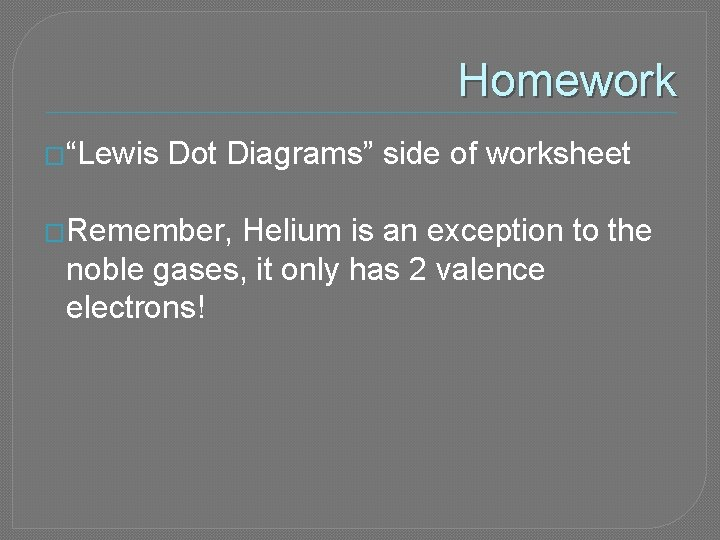Homework �“Lewis Dot Diagrams” side of worksheet �Remember, Helium is an exception to the noble gases, it only has 2 valence electrons!